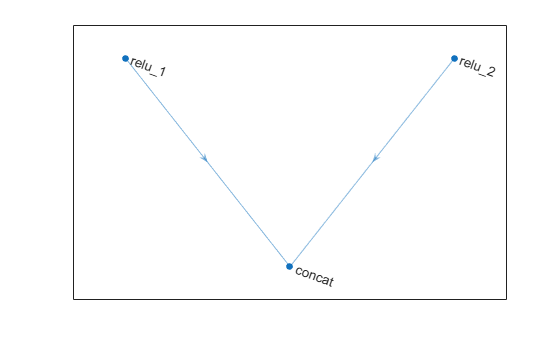# concatenationLayer

Concatenation layer

## Description

A concatenation layer takes inputs and concatenates them along a specified dimension. The inputs must have the same size in all dimensions except the concatenation dimension.

Specify the number of inputs to the layer when you create it. The inputs have the names `'in1','in2',...,'inN'`, where `N` is the number of inputs. Use the input names when connecting or disconnecting the layer by using `connectLayers` or `disconnectLayers`.

## Creation

### Syntax

``layer = concatenationLayer(dim,numInputs)``
``layer = concatenationLayer(dim,numInputs,'Name',name)``

### Description

example

````layer = concatenationLayer(dim,numInputs)` creates a concatenation layer that concatenates `numInputs` inputs along the specified dimension, `dim`. This function also sets the `Dim` and `NumInputs` properties.```
````layer = concatenationLayer(dim,numInputs,'Name',name)` also sets the `Name` property.```

## Properties

expand all

### Concatenation

Concatenation dimension, specified as a positive integer.

Example: `4`

### Layer

Layer name, specified as a character vector or a string scalar. For `Layer` array input, the `trainNetwork`, `assembleNetwork`, `layerGraph`, and `dlnetwork` functions automatically assign names to layers with `Name` set to `''`.

Data Types: `char` | `string`

Number of inputs to the layer, specified as a positive integer greater than or equal to 2.

The inputs have the names `'in1','in2',...,'inN'`, where `N` is `NumInputs`. For example, if `NumInputs` is 3, then the inputs have the names `'in1','in2'`, and `'in3'`. Use the input names when connecting or disconnecting the layer using the `connectLayers` or `disconnectLayers` functions.

Input names, specified as `{'in1','in2',...,'inN'}`, where `N` is the number of inputs of the layer.

Data Types: `cell`

Number of outputs of the layer. This layer has a single output only.

Data Types: `double`

Output names of the layer. This layer has a single output only.

Data Types: `cell`

## Examples

collapse all

Create a concatenation layer that concatenates two inputs along the fourth dimension (channels). Name the concatenation layer `'concat'`.

`concat = concatenationLayer(4,2,'Name','concat')`
```concat = ConcatenationLayer with properties: Name: 'concat' Dim: 4 NumInputs: 2 InputNames: {'in1' 'in2'} ```

Create two ReLU layers and connect them to the concatenation layer. The concatenation layer concatenates the outputs from the ReLU layers.

```relu_1 = reluLayer('Name','relu_1'); relu_2 = reluLayer('Name','relu_2'); lgraph = layerGraph(); lgraph = addLayers(lgraph, relu_1); lgraph = addLayers(lgraph, relu_2); lgraph = addLayers(lgraph, concat); lgraph = connectLayers(lgraph, 'relu_1', 'concat/in1'); lgraph = connectLayers(lgraph, 'relu_2', 'concat/in2'); plot(lgraph)```